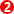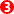﻿ General Design Information > Cam Mechanisms > Design Checks with the Cam-Data FB > Force Analysis: Generic Payload Signatures and Importance of Machine Speed.

# Force Analysis: Generic Payload Signatures and Importance of Machine Speed.

 IMPORTANT : Before looking at Contact-Force and Contact-Stress... When you want to analyze Cam-Force and Contact-Stress, it is important that the model represents the machine correctly. Even if the kinematics are correct, you may still need to reconfigure how to transmit the forces from the drive to the payload of the model.

'Payload' is the superposition (addition) of the different types of force that act between the cam and cam-follower.

'Payload Signature' is the payload plotted over one machine cycle or cam rotation.

Each 'Payload Type' has a function of machine-angle and of machine-speed.

ExampleSpring ForceViscosity, Air-CylinderInertia Forces'Power' stroke of an Engine

Note: * Friction-force is a function of the contact force, and, thus, it is not necessarily a constant value. Friction-forces are actually very difficult to calculate correctly. Thus, it is modelled as a 'Constant-Force' opposite to the direction of motion.

#### Importance of Machine Speed

In the case of the example below, the payload is a superposition of three load types:

Constant Force (Green), for example a Gravity, Air-Cylinder Spring-Pre-load

Displacement Force (Blue), for example a Spring Force

Acceleration Force (Magenta), for example an Inertia ForceCam forces at 'Low Speed'.

When we plot the force over one machine cycle, but at two different machine speeds:

Low-Speed:

Maximum payload is at the maximum displacement of the spring and cam-followerThe acceleration force is low.

High-Speed:

Maximum payload is at the large positive acceleration.

Minimum payload is a force that is less than 0Nat the large, negative acceleration (deceleration).

Note: The X–axis of the graph is 'Machine-Angle' not 'time'.

The actual time duration of the X–axis is less for the high-speed graph.